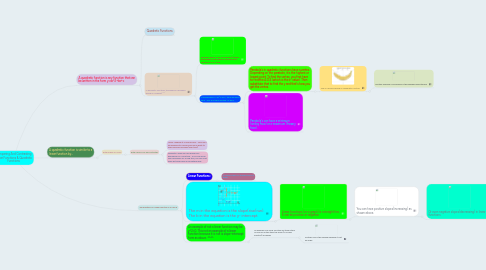# Comparing And Contrasting Linear Functions & Quadratic FunctionsAndrea Flick
Get Started. It's FreeComparing And Contrasting Linear Functions & Quadratic Functions## 1. A quadratic function is any function that can be written in the form y=ax^2+bx+c

### 1.2. In quadratic functions, the graph is a parabola which is U shaped^^^

1.2.1. Parabola's have an axis of symmetry (divides the parabola in two halves. The formula to find the A.O.S is -b/2a.

1.2.2. In the example: y=3x^2-6x+1, the 3 would be the a.  The -6 is the b and the 1 is the c.

1.2.2.1. Parabola's in quadratic functions have a vertex. Depending on the parabola, it is the highest or lowest point. To find the vertex, you first have to find the A.O.S (which is the x- value). Then substitute that to find the y and that's how you get the vertex.

1.2.2.1.1. This is a banana which is a quadratic function

1.2.2.2. Parabola's can have a minimum (smiley face) or a maximum (frowny face).

## 2. The equation of a linear function is y=mx=b

### 2.1. Linear Functions:

2.1.1. A function that is a straight line on a graph

### 2.2. The m in the equation is the slope(rise/run). The b in the equation is the y- intercept.

2.2.1. Linear functions (on a graph) is a straight line. It can be positive or negative

2.2.1.1. You can have positive slopes(increasing) as shown above.

2.2.1.1.1. Or even negative slopes(decreasing) in linear functions

### 2.3. An example of not a linear function may be y^2=0. This not an example of a linear function because it is not is slope-intercept form as above. ^^^^

2.3.1. An example of a linear function are these stairs. As you go up the stairs the slope (in a linear function) increases

2.3.1.1. Another one is this seesaw because it is at an angle

## 3. A quadratic function is similar to a linear function by...

### 3.1. Both graphs on a line

3.1.1. Both used in our daily activities

3.1.1.1. Linear: Walking at a normal pace.... since you are walking at a normal pace your ability to walk more will increase (over time)

3.1.1.2. Quadratic: When you are doing your homework for a long time... if you are doing your homework for a long time, you will most likely get bored and do something else